This book is archived and will be removed July 6, 2022. Please use the updated version.

The Nature of Light

# 4 Refraction

### Learning Objectives

By the end of this section, you will be able to:

• Describe how rays change direction upon entering a medium
• Apply the law of refraction in problem solving

You may often notice some odd things when looking into a fish tank. For example, you may see the same fish appearing to be in two different places ((Figure)). This happens because light coming from the fish to you changes direction when it leaves the tank, and in this case, it can travel two different paths to get to your eyes. The changing of a light ray’s direction (loosely called bending) when it passes through substances of different refractive indices is called refraction and is related to changes in the speed of light,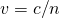. Refraction is responsible for a tremendous range of optical phenomena, from the action of lenses to data transmission through optical fibers.

(a) Looking at the fish tank as shown, we can see the same fish in two different locations, because light changes directions when it passes from water to air. In this case, the light can reach the observer by two different paths, so the fish seems to be in two different places. This bending of light is called refraction and is responsible for many optical phenomena. (b) This image shows refraction of light from a fish near the top of a fish tank.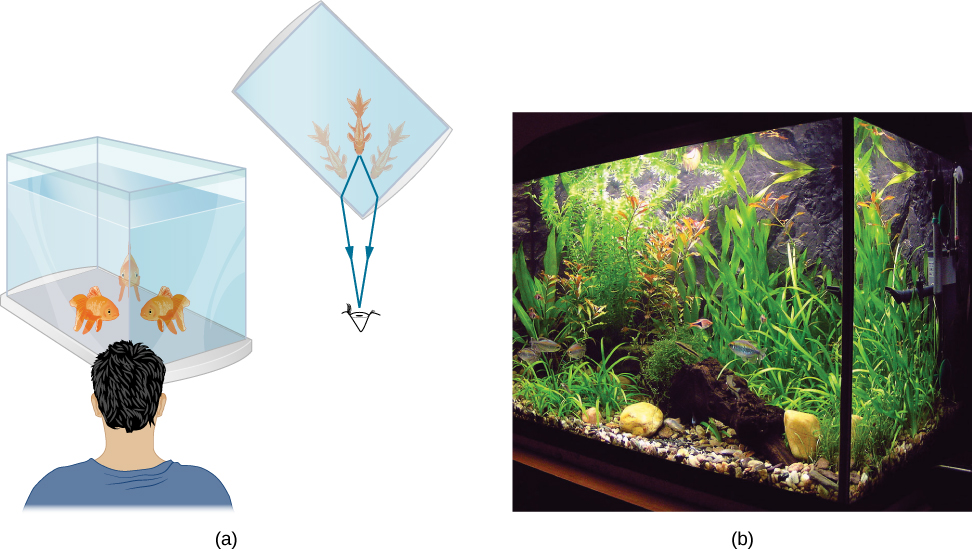(Figure) shows how a ray of light changes direction when it passes from one medium to another. As before, the angles are measured relative to a perpendicular to the surface at the point where the light ray crosses it. (Some of the incident light is reflected from the surface, but for now we concentrate on the light that is transmitted.) The change in direction of the light ray depends on the relative values of the indices of refraction (The Propagation of Light) of the two media involved. In the situations shown, medium 2 has a greater index of refraction than medium 1. Note that as shown in (Figure)(a), the direction of the ray moves closer to the perpendicular when it progresses from a medium with a lower index of refraction to one with a higher index of refraction. Conversely, as shown in (Figure)(b), the direction of the ray moves away from the perpendicular when it progresses from a medium with a higher index of refraction to one with a lower index of refraction. The path is exactly reversible.

The change in direction of a light ray depends on how the index of refraction changes when it crosses from one medium to another. In the situations shown here, the index of refraction is greater in medium 2 than in medium 1. (a) A ray of light moves closer to the perpendicular when entering a medium with a higher index of refraction. (b) A ray of light moves away from the perpendicular when entering a medium with a lower index of refraction.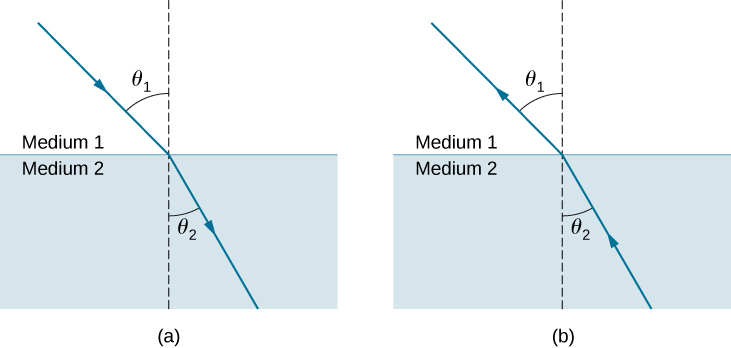The amount that a light ray changes its direction depends both on the incident angle and the amount that the speed changes. For a ray at a given incident angle, a large change in speed causes a large change in direction and thus a large change in angle. The exact mathematical relationship is the law of refraction, or Snell’s law, after the Dutch mathematician Willebrord Snell (1591–1626), who discovered it in 1621. The law of refraction is stated in equation form as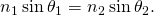Here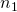and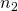are the indices of refraction for media 1 and 2, and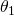and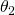are the angles between the rays and the perpendicular in media 1 and 2. The incoming ray is called the incident ray, the outgoing ray is called the refracted ray, and the associated angles are the incident angle and the refracted angle, respectively.

Snell’s experiments showed that the law of refraction is obeyed and that a characteristic index of refraction n could be assigned to a given medium and its value measured. Snell was not aware that the speed of light varied in different media, a key fact used when we derive the law of refraction theoretically using Huygens’s principle in Huygens’s Principle.

Determining the Index of Refraction Find the index of refraction for medium 2 in (Figure)(a), assuming medium 1 is air and given that the incident angle is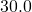and the angle of refraction is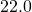.

Strategy The index of refraction for air is taken to be 1 in most cases (and up to four significant figures, it is 1.000). Thus,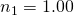here. From the given information,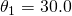and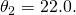With this information, the only unknown in Snell’s law is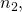so we can use Snell’s law to find it.

Solution From Snell’s law we have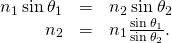Entering known values,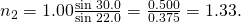Significance This is the index of refraction for water, and Snell could have determined it by measuring the angles and performing this calculation. He would then have found 1.33 to be the appropriate index of refraction for water in all other situations, such as when a ray passes from water to glass. Today, we can verify that the index of refraction is related to the speed of light in a medium by measuring that speed directly.

Explore bending of light between two media with different indices of refraction. Use the “Intro” simulation and see how changing from air to water to glass changes the bending angle. Use the protractor tool to measure the angles and see if you can recreate the configuration in (Figure). Also by measurement, confirm that the angle of reflection equals the angle of incidence.

A Larger Change in Direction Suppose that in a situation like that in (Figure), light goes from air to diamond and that the incident angle is. Calculate the angle of refractionin the diamond.

Strategy Again, the index of refraction for air is taken to be, and we are given. We can look up the index of refraction for diamond in (Figure), finding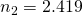. The only unknown in Snell’s law is, which we wish to determine.

Solution Solving Snell’s law for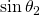yields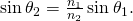Entering known values,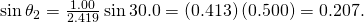The angle is thusSignificance For the sameangle of incidence, the angle of refraction in diamond is significantly smaller than in water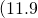rather than—see (Figure)). This means there is a larger change in direction in diamond. The cause of a large change in direction is a large change in the index of refraction (or speed). In general, the larger the change in speed, the greater the effect on the direction of the ray.

Check Your Understanding In (Figure), the solid with the next highest index of refraction after diamond is zircon. If the diamond in (Figure) were replaced with a piece of zircon, what would be the new angle of refraction?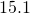### Summary

• The change of a light ray’s direction when it passes through variations in matter is called refraction.
• The law of refraction, also called Snell’s law, relates the indices of refraction for two media at an interface to the change in angle of a light ray passing through that interface.

### Conceptual Questions

Diffusion by reflection from a rough surface is described in this chapter. Light can also be diffused by refraction. Describe how this occurs in a specific situation, such as light interacting with crushed ice.

Will light change direction toward or away from the perpendicular when it goes from air to water? Water to glass? Glass to air?

“toward” when increasing n (air to water, water to glass); “away” when decreasing n (glass to air)

Explain why an object in water always appears to be at a depth shallower than it actually is?

Explain why a person’s legs appear very short when wading in a pool. Justify your explanation with a ray diagram showing the path of rays from the feet to the eye of an observer who is out of the water.

A ray from a leg emerges from water after refraction. The observer in air perceives an apparent location for the source, as if a ray traveled in a straight line. See the dashed ray below.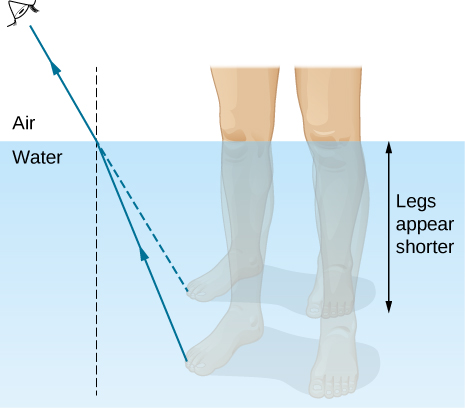Explain why an oar that is partially submerged in water appears bent.

### Problems

Unless otherwise specified, for problems 1 through 10, the indices of refraction of glass and water should be taken to be 1.50 and 1.333, respectively.

A light beam in air has an angle of incidence of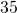at the surface of a glass plate. What are the angles of reflection and refraction?

A light beam in air is incident on the surface of a pond, making an angle of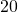with respect to the surface. What are the angles of reflection and refraction?

reflection,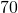; refraction,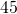When a light ray crosses from water into glass, it emerges at an angle of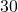with respect to the normal of the interface. What is its angle of incidence?

A pencil flashlight submerged in water sends a light beam toward the surface at an angle of incidence of. What is the angle of refraction in air?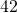Light rays from the Sun make aangle to the vertical when seen from below the surface of a body of water. At what angle above the horizon is the Sun?

The path of a light beam in air goes from an angle of incidence ofto an angle of refraction of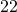when it enters a rectangular block of plastic. What is the index of refraction of the plastic?

1.53

A scuba diver training in a pool looks at his instructor as shown below. What angle does the ray from the instructor’s face make with the perpendicular to the water at the point where the ray enters? The angle between the ray in the water and the perpendicular to the water is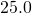.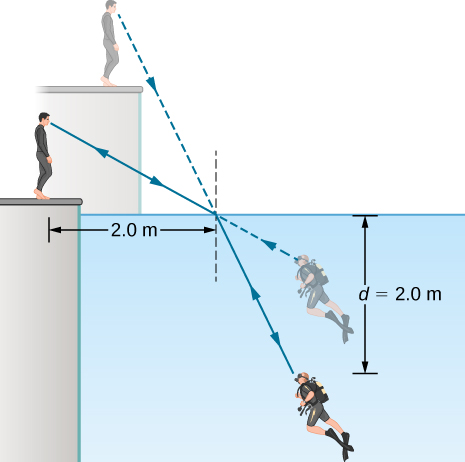(a) Using information in the preceding problem, find the height of the instructor’s head above the water, noting that you will first have to calculate the angle of incidence. (b) Find the apparent depth of the diver’s head below water as seen by the instructor.

a. 2.9 m; b. 1.4 m

### Glossary

law of refraction
when a light ray crosses from one medium to another, it changes direction by an amount that depends on the index of refraction of each medium and the sines of the angle of incidence and angle of refraction
refraction
changing of a light ray’s direction when it passes through variations in matter